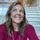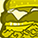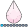## A method to speed up PROC PHREG when doing a Cox regression

Started ‎01-28-2015 by
Modified ‎01-28-2016 by
Views 6,686

# How to speed up PROC PHREG when doing a Cox regression

I would here like to show how you can speed up your PHREG when doing a Cox-regression. When you have either left-truncated survival times or if you have time-dependent effects the calculation time of PROC PHREG depends per default quadritic on the size of population. By doing an aggregation before using PROC PHREG or by using the FAST option introduced in SAS/STAT 14.1 the total calculation time can be reduced to depend linear with respect to size of studypopulation. This difference can be huge.

If there is no lefttruncation nor any timedependent variables then PHREG does a time-efficient estimation and the method explained in this article is in that case not a help.

Some changes are made in this article since it was published first time in 2015. FAST option was introduced in SAS/STAT 14.1 which in many cases makes the use of the shown macro obsolete. Also competing risk options are added to the macro which makes efficient aggregation when there are multiple eventtypes.

## Method

The first thing to do when speed up the Cox-regression is to collect the sufficient statistics in a dataset. The sufficient statisics in a Cox-regression is found be counting the numbers of persons at risk and the numbers of deaths at each timepoint where there is at least one death. These numbers should be calculated for each combination of covariates. Calculating these numbers can be done quite fast (linear with respect to population size). Using PHREG on the aggregated dataset is typical done very fast since the aggregated data often is relative small. This allows the use of Cox-regression analyzed with PHREG even on huge dataset with a huge number of deaths. - and it is easy!

The sufficient statistics has to take a form that can be analyzed by PROC PHREG. This can be done by creating a new variable "dummytime" which only can take the values 1 or 2. Persons  that have events get  dummytime=1 and those who are not events have dummytime=2, the latter are censored. Further, a weight variable counts how many persons each observation represents.

The original dataset can take the form like this

ID Entry Exit Covariate 1 Covariate 2 Event
1 0 4 A - 0
1 4 12 A + 1
2 0 5 B - 0
.. .. ..
.. .. ..

While the sufficient dataset can take a form like this

Time Covariate 1 Covariate 2 Dummytime weight
12 A + 1 1
12 A + 2 99
12 A - 2 100
12 A - 2 100
12 B + 2 100
12 B - 2 100
.. -- .. .. ..

Notice that the sufficient dataset contain a block of observations for each timepoint where at least one death is observed, and the timevariable says when it happened. By stratifying on the timevariable the likelihood will take a form of a product of terms from each distinct value of time. This gives exatly the same as in the original likelihood. I leave the details to the readers.

## Examles

The examples Works on a dataset "mydata", with a left-truncation time "entry", censoring or event-time "exit" and a censoring/event indicator "event". The explanatory variables is "covariate1-covariate4". In the attached macro the full examples are found below the macro-definition together with a datastep data simulate the data used in the examples.

## Example 1: A basic Cox regression

### The naive way

Using PROC PHREG in the naive way will look like this

``````PROC PHREG DATA=mydata;
CLASS  covariate1-covariate4;
MODEL (entry exit)*event(0)=covariate1-covariate4/ties=BRESLOW;
RUN;
``````

For just moderate size of the inputdataset, the calculation time can be too long for us who are impatient.

### The smart way

The attached macro can be used to calculate the sufficient statistics, afterwards PROC PHREG can be used on the aggregated data. This can look like this:

``````%coxaggregate(data=mydata,output=dataout,entry=entry,exit=exit,event=event,covariate=covariate1+covariate2+covariate3+covariate4)
PROC PHREG DATA=dataout NOSUMMARY;
CLASS covariate1-covariate4;
MODEL dummytime*dummytime(2)= covariate1-covariate4 /TIES=BRESLOW;
STRATA time ;
FREQ weight;
RUN;
``````

One should note the NOSUMMARY-option as this prevent summary statistics from eash strata to be printed. This is important, as the methods here use STRATA to distinguish riskset from each other.

### The smartest way

Using SAS/STAT 14.1 or newer it is possible to let the PHREG procedure do the aggregation. This is done with the FAST option

``PROC PHREG DATA=mydata FAST;  CLASS  covariate1-covariate4;  MODEL (entry exit)*event(0)=covariate1-covariate4/ties=BRESLOW;RUN;``

This will produce exactly same numbers as the naive approache since it is the same likelihood functions that is maximized. The above approach works only for the Breslow and Efron methods for handling ties (more than one event at same timepoint).

## Example 2: A Cox regression with a strata-effect, a by-variable and a weight variable.

This example is just a slightly extended version of the one in example 1. Here I introduce a strata variable, "stratavar", and I want to to the analyses separatly for the different values of the by-variable "byvar". Further, each observation is counted with the number specified in the weight variable.

### The naive way

Using PROC PHREG in the naive way will look like this

``````PROC PHREG DATA=mydata;
CLASS  covariate1-covariate4;
MODEL (entry exit)*event(0)=covariate1-covariate4/ties=BRESLOW;
STRATA stratavar;
BY  byvar;
WEIGHT weight;
RUN;
``````

For just moderate size of the inputdataset, the calculationtime can be too long for us who are impatient.

### The smart way

The numbers of persons at risk and numbers of events should be calculated separately for each combination of different values of byvariables and strata-variables. Therefore, these are used in the "by=" argument in the "%coxaggregate" macro. Afterwards, the byvariables are (as usual) added to the BY statement in PHREG and the strata variables are added to the STRATA statement together with the time variable created by the macro. The weight variable is used as an argument for the macro.

``````%coxaggregate(data=mydata,output=dataout,entry=entry,exit=exit,event=event,covariate=covariate1+covariate2+covariate3+covariate4,by=byvar+stratavar,weight=weight);
PROC PHREG DATA=dataout NOSUMMARY;
CLASS covariate1-covariate4
MODEL dummytime*dummytime(2)= covariate1-covariate4 /TIES=BRESLOW;
STRATA time stratavar ;
FREQ weight ;
BY byvar;
RUN;
``````

### The smartest way

A faster (slightly faster than the macro) is to use the FAST option.

``````PROC PHREG DATA=mydata FAST;
CLASS  covariate1-covariate4;
MODEL (entry exit)*event(0)=covariate1-covariate4/ties=BRESLOW;
STRATA stratavar;
BY  byvar;
WEIGHT weight;
RUN;
``````

### Example 3: An example with ties=discrete

If you better like to use the DISCRETE method for handling ties, the naive way to calculate the incidence ratios is with a program like this

### The naive way

``````PROC PHREG DATA=mydata;
CLASS  covariate1-covariate4;
MODEL (entry exit)*event(0)=covariate1-covariate4/ties=discrete;
RUN;
``````

PHREG can take vere long time to calculate the discrete version of the  likelihod. For testing purpose the used data should be kept small!

### The smart way

Again, the sufficient statistics has to be calculated as the first thing. Afterwards PHREG is used with ties=discrete

``````%coxaggregate(data=simulation,output=dataout,entry=entry,exit=exit,event=event,covariate=covariate1+covariate2+covariate3+covariate4);
proc phreg data=dataout nosummary;
class covariate1-covariate4/param=glm ;
model dummytime*dummytime(2)= covariate1-covariate4/rl type3(WALD ) ties=discrete;
STRATA time;
FREQ weight;
RUN;
``````

An alternative way to get same result is to aggregate data into an form on which conditional logistic regression can be used. This is done with "TYPE=LOGISTIC" when calling the %coxaggregate macro.

``````%coxaggregate(data=simulation,output=dataout,entry=entry,exit=exit,event=event,covariate=gruppe1+gruppe2,type=logistic);
proc logistic data=dataout desc;
class covariate1-covariate4/param=glm ;
model d/n=covariate1-covariate4;
strata time/nosummary;
run;
``````

## Example 4: Time dependent effects

The %coxaggregate-macro also work if there are timedependent covariates. Though, effects are only allowed to depend on the underlying timeaxis, not some other time found on individual level. Otherwise the aggregation can not be done. Lets say for instance that the effect of covariate1,  is allowed to change its value when the underlying time is 5. The value of covariate1 is assumed to be 0 or 1.

### The naive way

``````PROC PHREG DATA=mydata;
covariate1_1=(exit<=5)*covariate1;
covariate1_2=(exit>5)*covariate1;
MODEL (entry exit)*event(0)=covariate1_1 covariate1_2 /ties=BRESLOW;
RUN;
``````

### The smart way

By first calculating the sufficient statistics we can introduce the time-dependent effect as an interaction term between time and covariate1. This is easily done with a "*" in the model line. The HAZARDRATIO statement allow us to easily calculate the effect of covariate1 for each value of the time-variable.

``````%coxaggregate(data=mydata,output=dataout,entry=entry,exit=exit,event=event,covariate=covariate1)
DATA dataout;
SET dataout;
more5=(time>5);
RUN;

PROC PHREG DATA=dataout NOSUMMARY;
CLASS  covariate1 more5 /param=glm ;
MODEL dummytime*dummytime(2)=  covariate1*more5 /rl  TIES=BRESLOW;
HAZARDRATIO covariate1/ at( more5=ALL);
STRATA time ;
FREQ weight;
RUN;
``````

The FAST option will not work in case there are timedependent variables defined inside PHREG. If one will use the FAST option in an example like the one shown here, the dataset should then be splitted up in "constant pieces". That means one record with entry=0, exit=5, event=0, and an other record with entry=5 and exit and event dependent on how the individual terminates.

## Example 5: Competing risk

Assume wh have a competing risk model. That is, there is multiple eventtypes, and the eventtype is given by the variable "eventtype". Also, an important property of the model is that the risk time (entry and exit) is the same for all types of event. The input dataset can then be constructed as above with addition of the variable "eventtype".

 ID Entry Exit Covariate 1 Covariate 2 Event Eventtype 1 0 4 A - 0 . 1 4 12 A + 1 2 2 0 5 B - 0 .. .. .. .. .. ..

One way to analyze such data is to make one dataset for each eventtype, where event only occur if eventtype is the one to be studied, or alternatively the these dataset can be putted together in one dataset:

 ID Entry Exit Covariate 1 Covariate 2 Event Eventtype 1 0 4 A - 0 1 1 4 12 A + 0 1 2 0 5 B - 0 1 .. .. .. 1 .. .. .. 1 1 0 4 A - 0 2 1 4 12 A + 1 2 2 0 5 B - 0 2 .. .. .. 2 .. .. .. 2

and then analyzed "by eventtype".

``````proc phreg data=competingrisk_data nosummary;
class covariate1 covariate2/param=glm ;
model (entry exit)*event2(0)=covariate1 covariate2;
by eventtype;
hazardratio covariate1;
run;``````

## The smarter way

Instead of making the longer dataset it can be used more efficeantly that the risktime is the same for all eventtypes. That makes it possible to make the aggreation in just one single run. Here the opton "eventype "should be applied wto the macro.

``````%coxaggregate(data=simulation,output=dataout,entry=entry,exit=exit,event=event,covariate=covariate1,by=strata1,eventtype=eventtype);
proc phreg data=dataout nosummary;
class eventtype covariate1/param=glm;
model dummytime*dummytime(2)=eventtype*covariate1;
strata time strata1 eventtype ;
hazardratio covariate1/at(eventtype=all );
freq weight;
run;``````

The princip is, that the data should first be aggregated before it is made long

# Comparions of calculation time

As mentioned, the difference in calculation time can be huge. In the below table the calculation time for similar models calculated with both PHREG on the rawdata and on an aggregated data are shown for data of various sizes. The model used in this comparison  are similar to that shown in example 1, data are simulated with various number of observations.

number of observations PHREG (rawdata)

aggregation+

PHREG (aggregated data)

160 0.15 sec 0.15 sec
1600 3.7 sec 0.45 sec
16000 80 sec 1 sec
160000 660 sec 3.5 sec

## Conclusion

PROC PHREG is an efficient procedure to calculate estimates of rate-ratios. However, if one has large datasets one can increase the calculation time alot by aggregating data before using PHREG.This is great.. thank you for writing an article for Communities on SAS!Good piece! Grateful for your contribution. Keep 'em coming.Small changes are made: it was requested that the macro should be able to hande weights. So this is now added to an option to the coxaggregate-macro and also added to one of the examples in the article. Further, some more description is added in the top of the macro to better explain how it should be used.Jacob - is this not the macro that inspired the new FAST option in PROC PHREG that is first available in SAS/STAT 14.1 = SAS9.4 (TS1M3)?

The FAST option requires that one be using the model (t1, t2) counting-process syntax (typically for when there are time-dependent covariates) and gives performance improvement for large data sets.@OsoGris

Sorry my late response.

It is partly correct that this macro inspired the new FAST-option. I first did a macro which both made aggregation and the estimation of parameters, which I sent to the programmers in Cary. The macro shown here is only making the aggregation.

Comparing the calculation time between the macro above with phreg with FAST-option shows that "FAST" is fastest, but the difference is small. "FAST" is a very cool option I think!The method shown above is though still very usefull as works also when there are timedependent covariates, in which case FAST will not work, unless the timedependence is piecewise constant and the dataset then can be splitted up in "constant pieces".Just to clarify: The FAST option works with time-dependent covariates defined using the model (t1, t2) counting-process syntax and not with time-dependent covariates defined with programming statements inside the PROC PHREG step. Here is a blurb from the SAS/STAT 14.1 PHREG documentation:

FAST
uses an alternative algorithm to speed up the fitting of the Cox regression for a large data set that has the counting process style of input. Simonsen (2014<>) has demonstrated the efficiency of this algorithm when the data set contains a large number of observations and many distinct event times. The algorithm requires only one pass through the data to compute the Breslow or Efron partial log-likelihood function and the corresponding gradient and Hessian. PROC PHREG ignores the FAST option if you specify a TIES= option value other than BRESLOW or EFRON, or if you specify programming statements for time-varying covariates. You might not see much improvement in the optimization time if your data set has only a moderate number of observations.I would like to make some changes to the macro above, but unfortunately I have not rights to edit it.

1) When the "weight" statements are used when phreg is called after the macro, then it should be replaced by "freq" statements. This will not affect the estimates or standarderrors. But, if one for instance want "at risk" numbers from output statement, then this is only correct when freq is used (not when weight is used).

2) I made an improvement to the macro such that it can aggregate efficiently when there are multiple eventtypes in a competing risk model.

JacobI'm a bit late to the party, but this is fantastic!

Is there a faster way to run PHREG when testing the proportionality using time dependent covariates?

I find this is VERY time consuming in SAS, it would be great if there was a way to speed up the calculations.

e.g.

```proc phreg data=uis;
model time*censor(0) = age race treat site agesite age treatt;
aget = age*log(time);
treatt = treat*log(time);
run; ```@spud704. Yes you can do exactly this test much more timeefficient than the approach you suggest. Just aggregate on riskset and weight on the numbers at risk. Use the attached macro for the article.

In this little program piece I simulate a dataset with one covariate, such that there is a rateratio=1.5 for 1<=5 and rateratio=2 for t>5 (so not proportional hazard rates since the rateratio changes. Then I estimate first the overall rateratio, then the rateratio in the two intervals, and then the test for proportional hazardrate by constructing the log(time)xcovariate and test this variable out of the model. The same three models are then made on the aggregated dataset, - you will notice that the numbers are exactly the same, it just run much faster.

``````data simulation;
do covariate1=0 to 1;
do i=1 to 2000;
baseline=0.1;
rateratio1=1.5;
rateratio2=2;
t=rand('exponential',1/(baseline*rateratio1**(covariate1=1)));;
if t>5 then t=5+rand('exponential',1/(baseline*rateratio2**(covariate1=1)));;
entry=0;
event=1;
output;
end;
end;
drop i;
run;

proc phreg data=simulation nosummary;
class covariate1/param=glm ;
model (entry t)*event(0)=covariate1;
run;
proc phreg data=simulation nosummary;
covariate1_1=covariate1*(t<=5);
covariate1_2=covariate1*(t>5);
model (entry t)*event(0)=covariate1_1 covariate1_2/rl;
run;
proc phreg data=simulation nosummary;
logt=log(t)*covariate1;
class covariate1/param=glm ;
model (entry t)*event(0)=covariate1 logt/rl;
run;

*equivalent to:;
%coxaggregate(data=simulation,output=dataout,entry=entry,exit=t,event=event,covariate=covariate1);

proc phreg data=dataout nosummary;
class covariate1(ref="0") period/param=glm ;
model dummytime*dummytime(2)= covariate1 ;
hazardratio covariate1;
strata time ;
freq weight;
run;

data dataout;
set dataout;
period=(time<=5);
logt=log(time);
run;
proc phreg data=dataout nosummary;
class covariate1(ref="0") period/param=glm ;
model dummytime*dummytime(2)= covariate1*period ;
hazardratio covariate1/at(period=all);
strata time ;
freq weight;
run;
proc phreg data=dataout nosummary;
class covariate1(ref="0")/param=glm ;
model dummytime*dummytime(2)=covariate1 covariate1*logt/type3 ;
strata time ;
freq weight;
run;
``````

Good luck.

Version history
Last update:
‎01-28-2016 12:56 PM
Updated by:Contributors
Article Labels
Article Tags